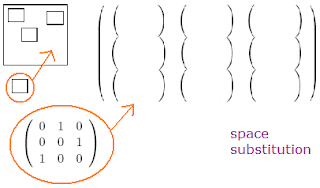occasional meanderings in physics' brave new world

Thursday, September 04, 2008

M Theory Lesson 221

Recall that a two dimensional kind of 1-operad is the little squares operad, which is like trees made of squares instead of points. Since matrices may be Chu spaces, we can also talk about the substitution of matrices into matrices as an operad of spaces. Note that the trivial case of the one dimensional number 1 acts like an operad of lattice squares, since matrices look like a square array of points.Restricting attention to matrices with entries 0 and 1 means assuming that all spaces belong to the category Chu(Set,2). In other words, a Chu space operad is like a topological space operad. However, the Chu space operations involve two different compositions, $+$ and $*$. For initial Chu spaces with $(p_1, s_1)$ and $(p_2, s_2)$ points and states, the new Chu space will have

$(p,s) = (p_1,s_1) + (p_2,s_2) = (p_1 + p_2, s_1 * s_2)$

and indeed the calculator tells us that the 0 for addition is the space $(0,1)$. For trees, these operations can be interpreted as in the graftingsThat is, in representing the initial spaces by 1-ordinal trees $p_i$ and $s_i$, the new Chu space is easily represented by a pair of 2-ordinal trees, where the number of leaves gives the final number of points and states. Compound additions of Chu spaces then result in higher dimensional ordinal trees. This is very different to the creation of associahedra, which are all labelled by 1-ordinal trees. However, selecting a sequence of Chu spaces with $0,1,2, \cdots$ points, say $\{ C_{p} \}$, results in well defined spaces

$C_{\sum n_i} \equiv C_{n_1} + C_{n_2} + \cdots + C_{n_k}$

What is an interesting such sequence? We could choose the quantum Fourier basis 1-circulant sequence, beginning with the Pauli swap matrix and then the $3 \times 3$ matrix $(231)$, interpreted as Set like Chu spaces! Then as the number of states is chosen to equal $p$, the space $C_{\sum n_i}$ will have $s = \prod n_i$ states. Restriction to a prime number of points, as in the MUB problem, results only in spaces with states given by prime factorisations for the integers.